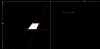# How do I get these two co-ordinates?

#### VeSo

##### MemberHeyall I'm using the draw_sprite_pos function to draw a sprite on an angle according to a top-central horizon point. With the help of image scaling I have determined the top left and right two circles and their respective x and y positions. (green) as well as the bottom blue circles y co-ordinates.

Could I possible use the lerp function to predict the final two x-cordinates based on input data from the top two corners? I have no idea.

Just to clarify, I'm trying to find the X location of both bottom corners of the square. The lines are perfect gradients.

Please help. I've been looking for a week now!#### chamaeleon

##### Member
Unless I'm missing something, just constant scaling?
Code:
``````green_x_offset = green_x - horiz_x
green_y_offset = green_y - horiz_y
blue_x = horiz_x + green_x_offset * ((green_y_offset + h)/green_y_offset)``````

#### DigitalRealms

##### Member
if you find side 'b', then you can use triangles similarity and find the rest

#### Attachments

• 9.6 KB Views: 8

#### chamaeleon

##### Member
if you find side 'b', then you can use triangles similarity and find the rest
The linear extrapolation applies to both. Just plug in the x and y for the two different green circles and out pops the x for the two different blue circles. No need to add complexity. Treat each line separately by in an identical manner, just wrtie a script that embodies the calculation and make two calls with the parameters required and assign the output to the corresponding x variable.

#### DigitalRealms

##### Member
The linear extrapolation applies to both. Just plug in the x and y for the two different green circles and out pops the x for the two different blue circles. No need to add complexity. Treat each line separately by in an identical manner, just wrtie a script that embodies the calculation and make two calls with the parameters required and assign the output to the corresponding x variable.
sure, you can use linear equation for that, which is lerp

#### chamaeleon

##### Member
sure, you can use linear equation for that, which is lerp
So with lerp
GML:
``````var green_y_offset = green_y - horiz_y;
var blue_x = lerp(horiz_x, green_x, (green_y_offset+h)/green_y_offset);``````